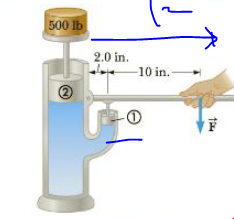Chapter 9, Problem 16P

Chapter
Section
Textbook Problem

Piston ① in Figure P9.16 has a diameter of 0.25 in.; piston ② has a diameter of 1.5 in. In the absence of friction, determine the force F → necessary to support the 500-lb weight.Figure P9.16

To determine
The force F that necessary to support the 500lb weight.

Explanation
At first, the force in the piston 1 by using Pascal’s principle as follows F1/A1=F2/A2F1=A1F2/A2 . It can be written as F1=(πd12/4πd22/4)F2=(d12/d22)F2 . The condition of static torque that passing through the left end of the jack handle, perpendicular to the page such that τ=0 will yield +F1l1Fl2=0F=(l1/l2)F1=(l1/l2)(d12/d22)F2 .

Given info: Diameters of pistons 1 and 2 are 0.25in and 1.5in , the weight of the object is 500lb , and the lengths are 12in and 2.0in

Still sussing out bartleby?

Check out a sample textbook solution.

See a sample solution

The Solution to Your Study Problems

Bartleby provides explanations to thousands of textbook problems written by our experts, many with advanced degrees!

Get Started